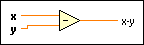# Subtract Function

## LabVIEW 2018 Help

Edition Date: March 2018
Part Number: 371361R-01
View Product InfoLabVIEW 2016 HelpLabVIEW 2017 HelpLabVIEW 2018 HelpLabVIEW 2019 HelpLabVIEW 2020 Help

Owning Palette: Numeric Functions

Requires: Base Development System

Computes the difference of the inputs.

If you wire two waveform values or two dynamic data type values to this function, error in and error out terminals appear on the function. Subtracting two time stamp values yields a numeric value (difference in time), and subtracting a numeric value from a time stamp value yields a time stamp. You cannot subtract a time stamp value from a numeric value. The dimensions of two matrices that you want to subtract must be the same. Otherwise, this function returns an empty matrix. The connector pane displays the default data types for this polymorphic function.x specifies the input value.

This input accepts the following data types:
• Number
• Timestamp
• Analog Waveform
This input also accepts an array or an array of clusters of the listed data types.y can be a scalar number, a fixed-point number, an array or cluster of numbers, an array of clusters of numbers, a time stamp, and so on.x-y is the difference between x and y.Note  You can manually configure this function to output data of a type you want. To specify the output data type, right-click the function and select Properties to display the Object Properties dialog box. On the Output Configuration page, click the Representation icon and select the data type you want. A blue coercion dot appears on the output terminal of the function to indicate that you have configured the output data type.

## Subtract Details

When you wire matrix data as an input to this function, a VI that includes subVIs that work with the matrix data type replaces the function. The resulting VI has the same icon but contains a matrix-specific algorithm. The node remains a VI if you disconnect the matrix from the input(s). Wire other data types as inputs to restore the original function. If you wire a data type to a function and that data type causes a basic math operation to fail, the function returns an empty matrix or NaN.

### Fixed-Point Details

If you wire fixed-point values to Numeric functions such as Add, Subtract, Multiply, and Square, the functions usually return values that do not lose any bits of word length. However, if the operation creates a value that exceeds the maximum word length that LabVIEW accepts, overflow or rounding conditions can occur. LabVIEW accepts a maximum word length of 64 bits. Use the Numeric Node Properties dialog box to configure how LabVIEW handles overflow or rounding of fixed-point data.

## Example

Refer to the Numeric Functions VI in the labview\examples\Numerics directory for an example of using the Subtract function.